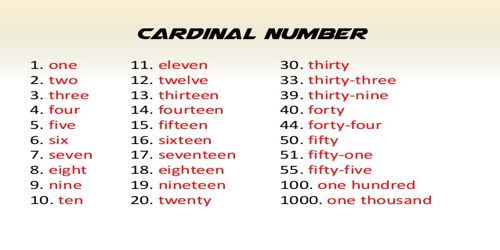Cardinal numbers (or cardinals) are numbers that say how many of something there are, such as one, two, three, four, five. A Cardinal Number is a natural number used for counting (e.g. 1, 2, 3 …).

It is the property that a mathematical set has in common with all sets that can be put in one-to-one correspondence with it. A cardinal number (also called “the cardinality”) is a type of number defined in such a way that any method of counting sets using it gives the same result. In fact, the cardinal numbers are obtained by collecting all ordinal numbers which are obtainable by counting a given set. The truth is neither of those possibilities answers the question as to what cardinal numbers are. Cardinal numbers are numbers that tell how many of something there are, such as one, two, three, four, or five.Cardinal number of a set

The number of distinct elements in a finite set is called its cardinal number. It is denoted as n(A) and read as ‘the number of elements of the set’.

For example:

(i) Set A = {2, 4, 5, 9, 15} has 5 elements.

Therefore, the cardinal number of set A = 5. So, it is denoted as n(A) = 5.

(ii) Set B = {w, x, y, z} has 4 elements.

Therefore, the cardinal number of set B = 4. So, it is denoted as n(B) = 4.

(iii) Set C = {Florida, New York, California} has 3 elements.

Therefore, the cardinal number of set C = 3. So, it is denoted as n(C) = 3.

(iv) Set D = {3, 3, 5, 6, 7, 7, 9} has 5 element.

Therefore, the cardinal number of set D = 5. So, it is denoted as n(D) = 5.

Though not all style guides agree, a common rule is that cardinal numbers one through nine are spelled out in an essay or article, while numbers 10 and above are written in figures. An alternative rule is to spell out numbers of one or two words (such as two and two million), and use figures for numbers that require more than two words to spell out (such as 214 and 1,412).

In either case, numbers that begin a sentence should be written out as words.

Regardless of which rule you choose to follow, exceptions are made for dates, decimals, fractions, percentages, scores, exact sums of money, and pages–all of which are generally written in figures. In business writing and technical writing, figures are used in nearly all cases.